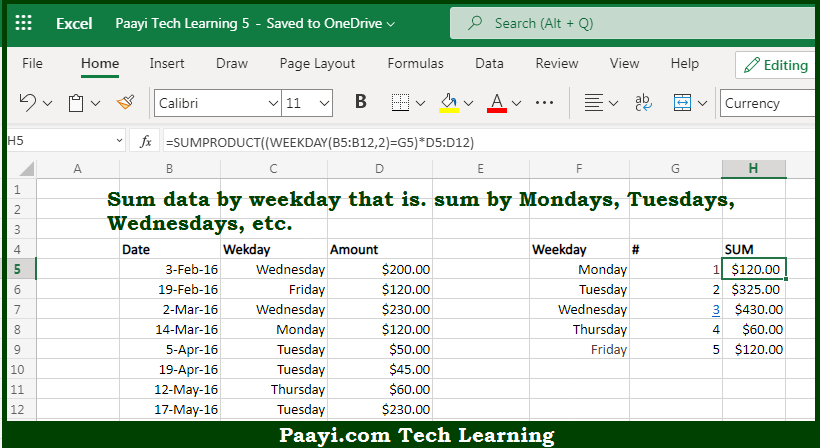# Learn How to SUM By Weekday in Microsoft Excel

Written by | 0 Comments | 617 Views

In this article, you will learn how to COUNT various things in Microsoft Excel using a single/combination(s) of functions. You will also know how to SUM By Weekday and see the generic formula.

SUM By Weekday in Microsoft Excel

The main purpose of this formula is to sum by weekday. Here we will learn how to sum by provided weekday in the workbook in Microsoft Excel. That implies, with the help of a formula based on the SUMPRODUCT function you can able sum by weekday, which means Monday, Tuesday, Wednesday, etc. So, with the help of this formula, you can able to sum by provided weekday in the workbook in Microsoft Excel.

General Formula to SUM By Weekday

=SUMPRODUCT((WEEKDAY(dates)=day_num)*values)

The Explanation for the SUM By WeekdaySo we know that with the help of the given formula above you can able to sum by weekday. Here we will learn how to sum by provided weekday in the workbook in Microsoft Excel. As you may get surprised why we aren't using the SUMIF or SUMIFS function in this example. That appears to be the obvious way to sum by the days of the week. Although, without adding a helper column with a weekday value, there is no way to create a criterion for SUMIF that takes into account the weekday. It should be noted that we are using the efficient SUMPRODUCT function, which handles arrays gracefully without requiring the use of Control + Shift + Enter. So, with the help of this formula, you can able to sum by provided weekday in the workbook in Microsoft Excel.